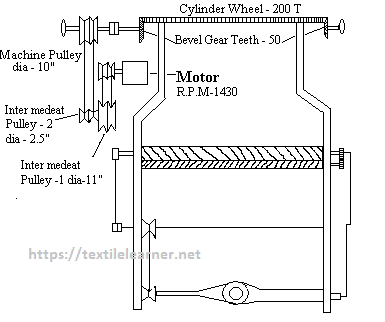# Production Calculation of Single Truck Single Jersey Circular Knitting Machine

Name of the experiment:
Study on the production calculation of single truck single jersey circular knitting machine.

Introduction:
Production of Single Jersey Single Truck Circular Knitting Machine means the length or weight of knitted fabrics produce per shift. A single jersey circular knitting machine’s production depends on it’s no. of needle, cylinder dia, motor r.p.m. etc.

Objects:

1. To know about the gearing diagram of the machine.
2. To make idea about the production of this types of this machine.

Machine Specification:

• Motor RPM = 1430
• Motor Pulley Dia = 2”
• Intermediate Pulley (1) Dia = 11”
• Intermediate Pulley (2) Dia = 2.5”
• Machine Pulley Dia = 10”
• Bevel Gear Teeth = 50T
• Cylinder Wheel Teeth = 200T
• Dia of the Cylinder = 19”
• No of Feeder = 24
• Machine Gauge = 22
• Overall Efficiency = 60%

Gearing diagram of single jersey single truck circular knitting machine:Fig: Gearing diagram of single jersey single truck circular knitting machine

Production Calculation:

………………CPM
Theory = —————-
………………CPI

CPM = Course Per minute
CPI = Course Per Inch

CPM = Cylinder RPM x No of Feeder

Cylinder RPM = Motor RPM x (2/11) x (2.5/10) x (50/200)
= 1430 x (2/11) x (2.5/10) x (50/200)
= 16.25

CPM = 16.25*24
= 390

Suppose CPI = 28

Production = 390/28 inch/min
= (390 x 60 x 8 x 0.91)/(28 x 36) meter/shift
= 169 meter/shift

Production Calculation in Weight:

CPM = RPM x No of Feeder x (π x d x Gauge of the machine x L)
= (16.25 x 24 x 3.1416 x 19” x 22 x 3mm x 60 x 8 x 0.6) / 1000 meter/shift
= 442478

Where,

D = Dia of the cylinder = 19”
L = Loop Length = 3 mm
Π = Constant = 3.1416

Let, count of the yarn is 20’s (English Count)
=> 840 x 20 x 0.91 meter = 0.453 kg
=> 442478 = (0.453 x 442478)/(840 x 20 x 0.91) kg
= 13.11 kg

Where,

0.91 yd = 1 meter
0.453 = 1 Pound

Production of the Single Jersey Circular Knitting Machine is 13.11 Kg Per Shift

Conclusion:
Single Jersey Circular knitting machine is primary or basic machine in the knitting technology. By this practical I came to know about the procedure of calculation the production of this type of circular knitting machine. I may use this experience in my practical job life.

You may also like: